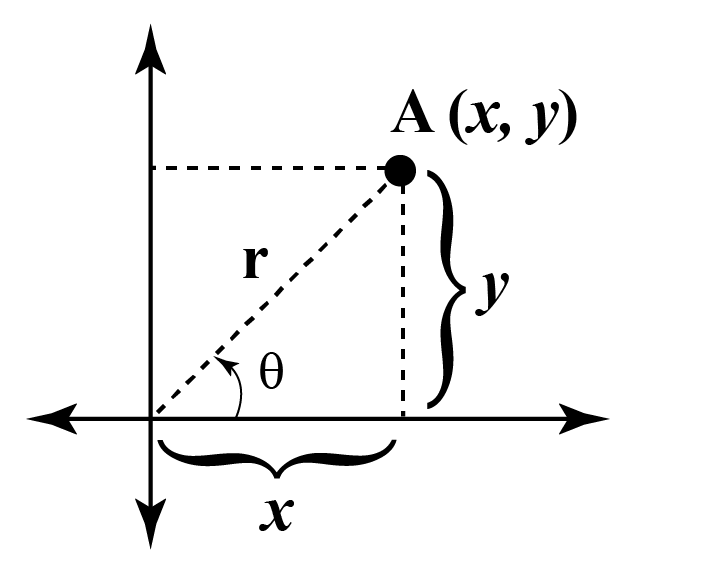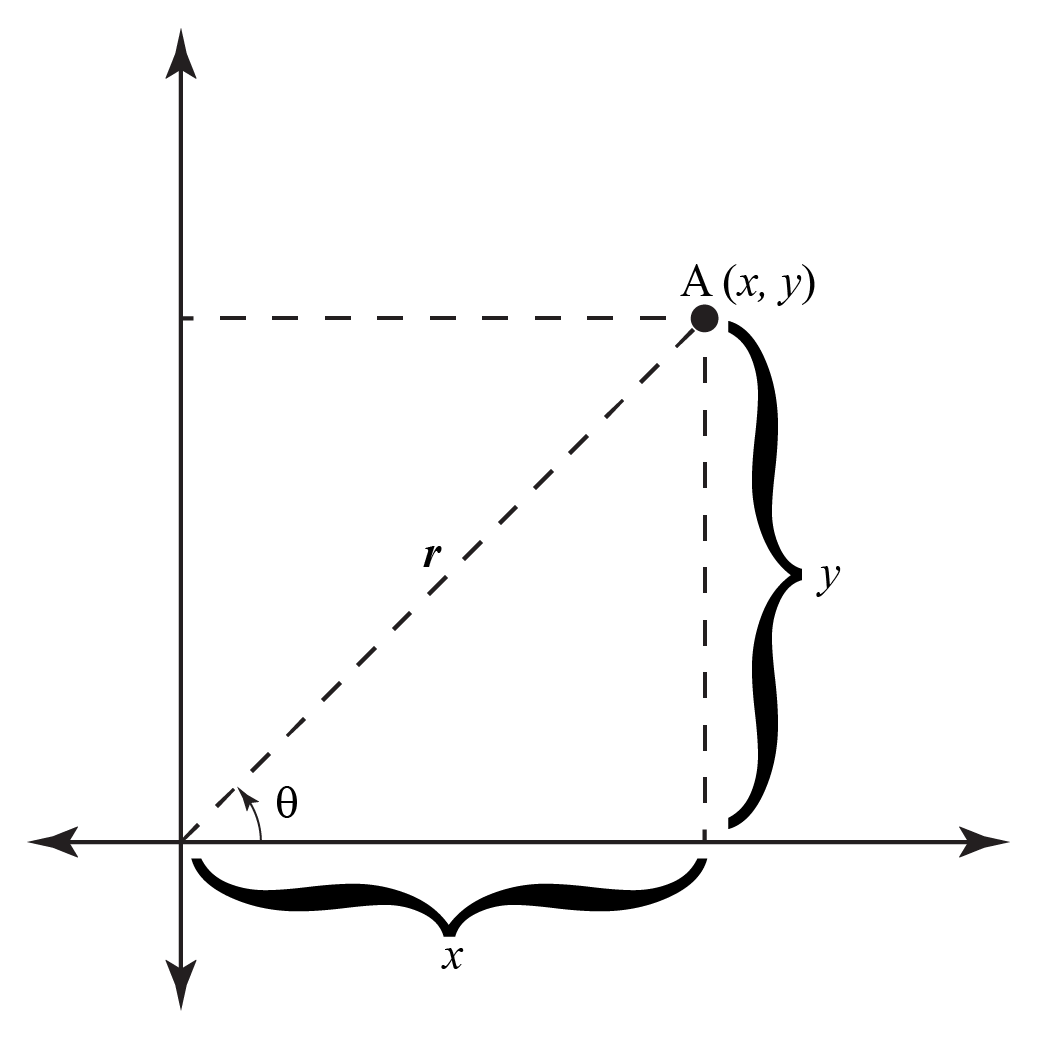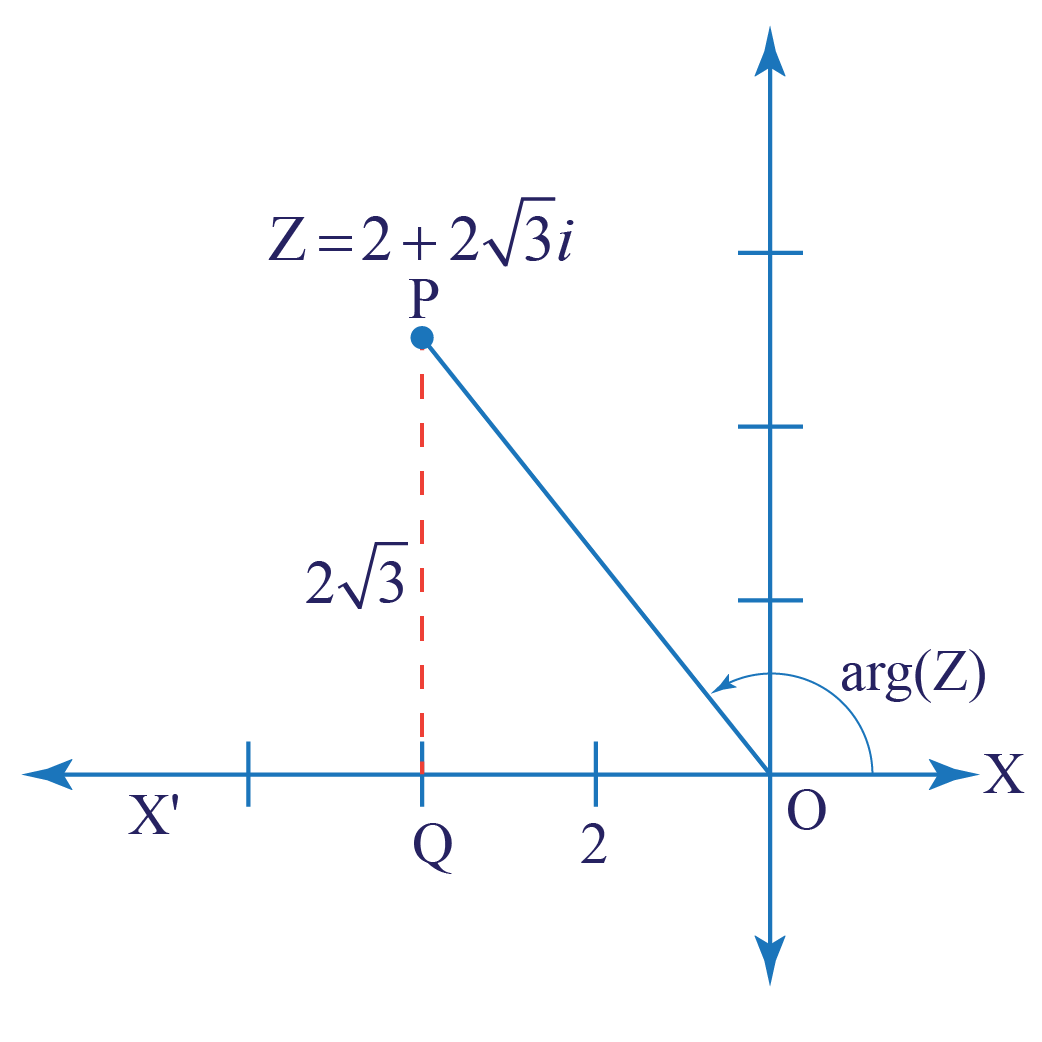# Polar Form of Complex Numbers

Polar Form of Complex Numbers
Go back to  'Complex Numbers'

The polar form of a complex number is another way of representing complex numbers.

The form z=a+bi is the rectangular form of a complex number.

Rectangular coordinates, also known as Cartesian coordinates were first given by Rene Descartes in the 17th century. It is said Sir Isaac Newton was the one who developed 10 different coordinate systems, one among them being the polar coordinate system.

In this mini lesson, we will get an overview of representing complex numbers, magnitude of complex numbers, argument of the complex number, modulus of the complex number, r signifies absolute value, and magnitude and argument.

Now, look at the figure below and try to identify the rectangular coordinates and the polar coordinates.## What Do You Mean by Polar Form of Complex Numbers?

In polar form, complex numbers are represented as the combination of r & θ, modulus and argument.

The polar form is represented with the help of polar coordinates of real and imaginary numbers in the coordinate system.

Let's say for a two-dimensional system:• The horizontal and vertical axis are the real axis and the imaginary axis.
•  r - the length of the vector and θ - the angle made with the real axis, are the real and complex components.
• There is a point $$A$$ with coordinates ($$x, y$$).
• The distance from the origin (0,0) to the point $$A$$ is given as $$r$$.
• The line joining the origin to the point $$A$$ makes an angle $$\theta$$ with the positive $$x$$-axis.
• The polar coordinates are given as ($$r, \theta$$) and rectangular coordinates are given as ($$x, y$$).

### Equation of Polar Form of Complex Numbers

$$\mathrm{z}=r(\cos \theta+i \sin \theta)$$

### Components of Polar Form Equation

In the case of a complex number

• r signifies absolute value or represents the modulus of the complex number.
• Angle θ is called the argument of the complex number.

## How Do You Represent Complex Numbers in Polar Form?

We write complex numbers in terms of a distance from the origin and a direction (or angle) from the positive horizontal axis.

Polar coordinates are expressed as ($$r, \theta$$)

For a complex number z=a+bi

Polar form is given by:

$$z=r(\cos \theta+i \sin \theta)$$

where

$$r=|z|=\sqrt{a^{2}+b^{2}}$$

$$a=r \cos \theta \text { and } b=r \sin \theta$$

$$\theta=\tan ^{-1}(y / x) \text { for } x>0$$

$$\theta=\tan ^{-1}(y / x)+180^{\circ} \text { for } x<0$$Tips and Tricks
• The values of polar coordinates and rectangular coordinates depend on each other. If we know any two values, the remaining two values can be found easily using the relation established between them.
• The conversion formulas for rectangular to polar coordinates are given as $$r = \sqrt{x^2 + y^2}$$ and $$\theta = \tan^{-1}(\frac{y}{x})$$.
• Take a look at the coordinate planes to know more.

## How to Convert Complex Numbers to Polar Form?

Consider the complex number $$z = - 2 + 2\sqrt 3 i$$, and determine its magnitude and argument. We note that z lies in the second quadrant, as shown below:Using Pythagoras Theorem, the distance of z from the origin, or the magnitude of z, is

$\left| z \right| = \sqrt {{{\left( { - 2} \right)}^2} + {{\left( {2\sqrt 3 } \right)}^2}} = \sqrt {16} = 4$

Now, let us calculate the angle between the line segment joining the origin to z (OP) and the positive real direction (ray OX).

Note that the angle POX' is

$\begin{array}{l}{\tan ^{ - 1}}\left( {\frac{{PQ}}{{OQ}}} \right) = {\tan ^{ - 1}}\left( {\frac{{2\sqrt 3 }}{2}} \right) = {\tan ^{ - 1}}\left( {\sqrt 3 } \right)\\ \qquad\qquad\qquad\qquad\qquad\;\;\,\,\,\,\,\,\,\,\,\, = {60^0}\end{array}$

Thus, the argument of z (which is the angle POX) is

$\arg \left( z \right) = {180^0} - {60^0} = {120^0}$

So, the polar form will be 4(cos120° + i sin120°)

## Interactive Questions

Here are a few activities for you to practice.

Select/Type your answer and click the "Check Answer" button to see the result.Challenging Questions
• Determine the value of arg(z,) given that z = 1-i?

## Let's Summarize

We hope you enjoyed learning about Polar Form of Complex Numbers with the simulations and practice questions. Now you will be able to easily solve problems on Polar Form of Complex Numbers in math with multiple math examples you learned today.

## About Cuemath

At Cuemath, our team of math experts is dedicated to making learning fun for our favorite readers, the students!

Through an interactive and engaging learning-teaching-learning approach, the teachers explore all angles of a topic.

Be it worksheets, online classes, doubt sessions, or any other form of relation, it’s the logical thinking and smart learning approach that we, at Cuemath, believe in.

## Frequently Asked Questions (FAQs)

### 1. How do you convert polar form of complex number to rectangular form?

The conversion formulas for polar to rectangular coordinates are given as:

$$x = r\cos\theta$$

$$y = r\sin\theta$$

### 2. What is the argument in polar form of complex number?

The angle formed between the positive x axis and the line joining a point with coordinates(x, y) to the origin is called the argument of the complex number.

### 3. What is polar and rectangular form?

The polar form of a complex number is z=r(cosθ+isinθ), whereas rectangular form is z=a+bi

### 4. What is the difference between argument and principal argument?

The argument is generally represented as (2nπ + θ), where n is an integer whereas, the value of principal argument is such that -π < θ < π.

### 5. Can argument of a complex number be negative?

Yes, the argument of a complex number can be negative, such as for -5+3i.

### 6. What is the argument of Z?

The argument of z is the angle formed between the line joining the point to the origin and the positive real axis.

### 7. What is the argument of 0?

Either undefined, or any real number is an argument of 0

### 8. Does magnitude and modulus mean the same?

The magnitude is also called the modulus.

### 9. How are magnitude and argument related?

The length of the line segment that is real axis is called the modulus of the complex number |z|.

The angle measured from the positive real axis to the line segment is called the argument of the complex number, arg(z).

More Important Topics
Numbers
Algebra
Geometry
Measurement
Money
Data
Trigonometry
Calculus